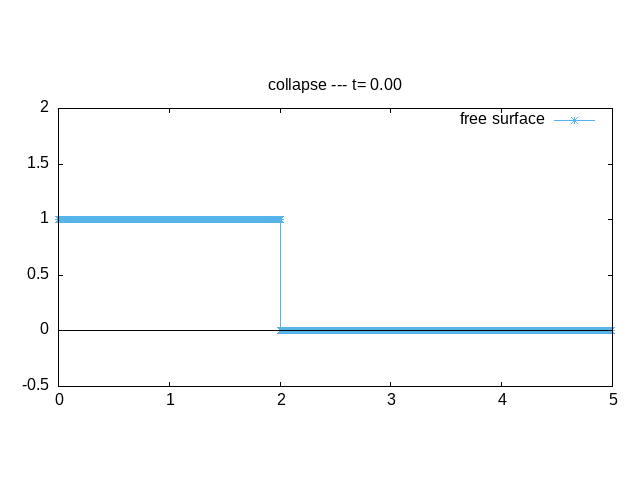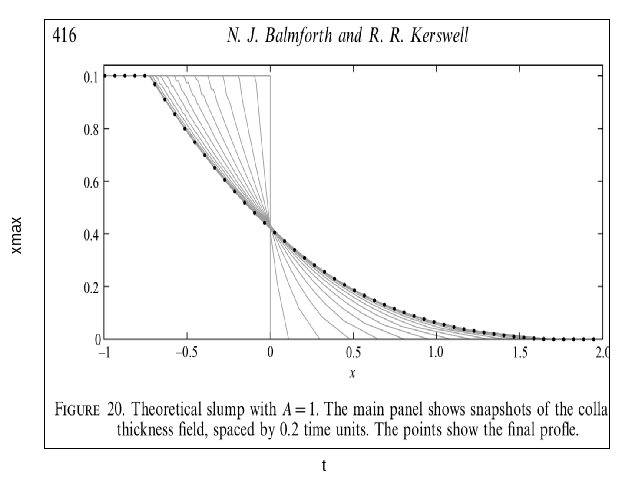# Collapse of granular heap

## Problem

This is the problem of the collapse of a initial rectangular heap of grains. It is a kind of Dam Break problem.animation of the collapse

## Equations

We compare the contact dynamic solution of granular collapse with “Shallow Water” or “Savage Hutter equations” or “depth averaged equations” or “Saint Venant” simplified equations with a basal friction \mu(I)P. Here P=\rho gh and I= 5./2.d (u/h)(g h)^{-1/2} . The system is \displaystyle \left\{\begin{array}{l} \frac{\partial }{\partial t} h \; +\; \frac{\partial }{\partial x} uh=s\\ \frac{\partial }{\partial t} hu + \frac{\partial }{\partial x} \dfrac{(hu)^2}{h} + \frac{\partial }{\partial x}g\dfrac{h^2}{2} = - gh \frac{\partial }{\partial x} Z-\mu g h\frac{u}{|u|} \end{array}\right.

Some pluviation s \ne 0 will be added as suggested in Larieu et all. (but is not yet implemented: here s = 0)

We solve the problem by splitting, first (note that we use Q=hu) \displaystyle \frac{h^*-h^n}{\Delta t} + \frac{\partial Q^n}{\partial x} =0, \text{ and } \frac{Q^*-Q^n}{\Delta t}+ \frac{\partial }{\partial x}\frac{Q^n}{h^n} + \frac{\partial }{\partial x}g\dfrac{h^{n2}}{2} = - g \frac{\partial }{\partial x} Z

is solved with http://basilisk.fr/src/saint-venant.h.

Second, (h is simplified) \displaystyle \frac{u^{n+1}-u^*}{\Delta t} =- \mu g \frac{u}{|u|} is solved.

## Code

#include "grid/multigrid1D.h"
#include "saint-venant.h"

The domain is 5 long, the height is the unit of length. The problem is without dimensions. The problem is solved in one dimension.

Wall symmetry at the left Neumann conditions at the exit

u.n[left] = dirichlet(0);
h[left] = neumann(0);
u.n[right] = neumann(0);
h[right] = neumann(0);

Main and parameters

//int source;
double Ltas,Htas,tmax;
int main()
{
X0 = 0.;
L0 = 7;
G = 1.;
N = 1024;
//  source = 0;
Htas = 1;
Ltas = 2.;
tmax=6;
DT=0.001;
run();
}

The initial conditions are a given heap of length L_{tas} (the double by symetry) of height H_{tas}. to left.

event init (i = 0){
double h0;

initial heap

    h0=Htas;//0.1*Htas*source + (1.-source)*Htas;
foreach(){
zb[] = 0;
h[] = (x < Ltas) ? h0 : 0;
u.x[]= 0;}
}

## Friction

We use a simple implicit scheme to implement coulomb bottom friction i.e. \frac{d\mathbf{u}}{dt}=-\mu g \frac{\mathbf{u}}{|\mathbf{u}|} with \mu fonction of a mean I (written with Q/h^{3/2}).

Of course we have splitted, first the Rieman Problem, second the friction problem.

• first we tried (as in viscous or turbulent cases) a semi implicit discretisation like: u(t+\Delta t) = u(t ) -\mu g (\Delta t) \frac{u(t+\Delta t) }{u(t ) } to obtain \displaystyle u(t+\Delta t) = \frac{u(t)}{1 + \mu g (\Delta t) \frac{1 }{u(t)}}

• finaly (see granular sand glass with friction) we noticed that there is an exact solution of this splited problem, defining the norm of velocity U=|\mathbf{u}| and \overrightarrow{T}=\frac{\mathbf{u}}{|\mathbf{u}|} the equation \frac{d (U \overrightarrow{T})}{dt}=-\mu g \overrightarrow{T} is solved explicitely for t'>t, obviously, as \frac{d \overrightarrow{T}}{ds}=\frac{\overrightarrow{N}}{R} we have just to solve: \displaystyle \frac{d U}{dt}=-\mu g it is linear and the solution is U(t')=U(t)- \mu g (t'-t) down to stop, so: \displaystyle U(t+\Delta t) = max(U(t) - \mu \Delta t g,0) This gives a real stop.

If \mu is function of a mean I across the layer, this mean I is obtained for a Bagnold profile as (2/5)(d/h)Q/\sqrt{g h } and \mu(I)=\mu_0 +\Delta \mu I/(I+I_0)

event coulomb_friction (i++) {
double In,nbrg=32.,eps=0.00000,mu,U,tauY=.001;
foreach() {
U=norm(u);
In=(1./nbrg)*5./2.*U/pow(h[]+eps,1.5);
mu = (0.4 + 0.28*In/(0.4+In)) ;

// mu = (0.4 + 0.26*exp(-0.136/In));
//   mu=.4;//.4; // to tes Kerswell
// obsolete
//    ff = U > 0 ? (1. +  dt *mu*G/U) : HUGE ;
//    foreach_dimension()
//      u.x[] /= ff;
// new and better
if(U>0){
foreach_dimension()
u.x[] = max(U -dt *( mu * G + tauY/h[]),0)*u.x[]/U;}
}
boundary ({u.x});
}

## output

event outputfront (t += .1 ) {
double  xf=0,xe=0;
static FILE * ff = fopen("x.txt", "w");

tracking the front and the end of the heap

    foreach(){
xf = h[] > 1e-20 ?  max(xf,x) :  xf ;
xe = h[] > 1e-20 ?  min(xe,x) :  xe ;
}
fprintf (ff, "%g %g %g \n", t, xf , xe);
}

event output (t += .01; t < tmax) {
static FILE * fp = popen ("gnuplot -persist 2> /dev/null", "w");
#ifdef gnuX

#else
if(t==0) fprintf (fp,"set term gif animate;set output 'animate.gif';set size ratio .5\n");
#endif
fprintf (fp,"set title ' collapse --- t= %.2lf '\n"
"p[0:5][-.5:2]  '-' u 1:($2) t'free surface' w lp lt 3,0 not w l linec -1\n",t); foreach() fprintf (fp, "%g %g \n", x, h[]); fprintf (fp,"e\n\n"); } event outputlog (t += 1; t < tmax) { foreach() fprintf (stderr, "%g %g %g \n", x, h[], t); fprintf (stderr, "\n"); } # Results to run and plot with gnuplot during the run (note the -DgnuX) qcc -g -O2 -DTRASH=1 -Wall -DgnuX=1 -o savagestaron savagestaron.c -lm with make  make savagestaron.tst;make savagestaron/plots; make savagestaron.c.html source ../c2html.sh savagestaron plot with gnuplot of the results, dynamics is not very good, but deposit is not so bad. set xlabel 'x' set ylabel 'h' set xlabel "x" set ylabel "h(x,t)" d=0.005 h0=0.149 p[0:6][0:1.5]'../../granular_column/ShapeTime.A-01.dat' u (($1*d)/h0):(($2*d)/h0) t'DCM'w l,'../savagestaron/log' w l t 'h'Fluid depth profile, Contact Dynamics vs Savage Hutter (script) Comparison with Balmforth & Kerswell 05 for the runout proposed by Kerswell 05 u = \frac{dx}{dt}=2 - \mu t so that x = 2 (t - \mu t^2/4) and t_{max} =\frac{2}{\mu} x_{max} =\frac{2}{\mu}  reset set xlabel "t" set ylabel "xmax" mu =.4 set key bottom p [][0:]'x.txt' u ($1):(($2-2)) t' calc',(x*(2-x*mu/2))*(x<2/mu?1:NaN) t'caract pred.'(script) Comparison with Balmforth & Kerswell 05 who rescaled to have a canonical problem:  set term png ; set output 'bk.png'; Xc=520 L1=907-Xc H1=640-185 Yc=185 mu=0.4 unset tics set key center top plot [0:1300] '../Img/Balmforth_Kerswell05.png' binary filetype=png with rgbimage not,\ 'log' u (Xc+($1-2)*L1*mu):(Yc+\$2*(H1)) w l notcmp B&K 05 (script)

## Bibliography

• Larrieu Staron Hinch “Raining into shallow water as a description of the collapse of a column of grains” J. Fluid Mech. (2006), vol. 554, pp. 259–270.

• Balmforth & Kerswell 05 “Granular collapse in two dimensions” J. Fluid Mech. (2005), vol. 538, pp. 399–428

• R. R. Kerswell Dam break with Coulomb friction: “A model for granular slumping?” PHYSICS OF FLUIDS 17, 057101 2005

v1: Montpellier 11 juillet 15